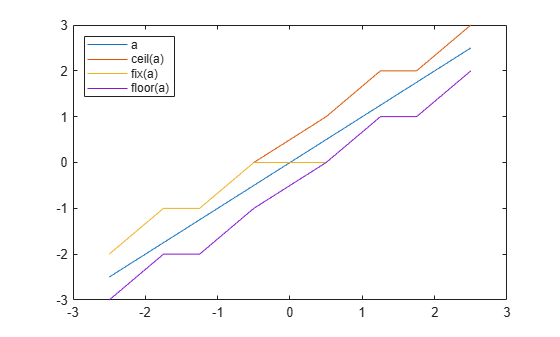ceil

Rounds toward positive infinity

Description

example

y = ceil(a) rounds fi object a to the nearest integer in the direction of positive infinity and returns the result in fi object y.

Examples

collapse all

The following example demonstrates how the ceil function affects the numerictype properties of a signed fi object with a word length of 8 and a fraction length of 3.

a = fi(pi,1,8,3)
a =
3.1250

DataTypeMode: Fixed-point: binary point scaling
Signedness: Signed
WordLength: 8
FractionLength: 3
y = ceil(a)
y =
4

DataTypeMode: Fixed-point: binary point scaling
Signedness: Signed
WordLength: 6
FractionLength: 0

The following example demonstrates how the ceil function affects the numerictype properties of a signed fi object with a word length of 8 and a fraction length of 12.

a = fi(0.025,1,8,12)
a =
0.0249

DataTypeMode: Fixed-point: binary point scaling
Signedness: Signed
WordLength: 8
FractionLength: 12
y = ceil(a)
y =
1

DataTypeMode: Fixed-point: binary point scaling
Signedness: Signed
WordLength: 2
FractionLength: 0

The functions ceil, fix, and floor differ in the way they round fi objects:

• The ceil function rounds values to the nearest integer toward positive infinity.

• The fix function rounds values to the nearest integer toward zero.

• The floor function rounds values to the nearest integer toward negative infinity.

This example illustrates these differences for a given fi input object a.

a = fi([-2.5,-1.75,-1.25,-0.5,0.5,1.25,1.75,2.5]');
y = [a ceil(a) fix(a) floor(a)]
y =
-2.5000   -2.0000   -2.0000   -3.0000
-1.7500   -1.0000   -1.0000   -2.0000
-1.2500   -1.0000   -1.0000   -2.0000
-0.5000         0         0   -1.0000
0.5000    1.0000         0         0
1.2500    2.0000    1.0000    1.0000
1.7500    2.0000    1.0000    1.0000
2.5000    3.0000    2.0000    2.0000

DataTypeMode: Fixed-point: binary point scaling
Signedness: Signed
WordLength: 16
FractionLength: 13
plot(a,y); legend('a','ceil(a)','fix(a)','floor(a)','location','NW');Input Arguments

collapse all

Input fi array, specified as scalar, vector, matrix, or multidimensional array.

For complex fi objects, the imaginary and real parts are rounded independently.

ceil does not support fi objects with nontrivial slope and bias scaling. Slope and bias scaling is trivial when the slope is an integer power of 2 and the bias is 0.

Data Types: fi
Complex Number Support: Yes

Algorithms

• y and a have the same fimath object and DataType property.

• When the DataType property of a is single, double, or boolean, the numerictype of y is the same as that of a.

• When the fraction length of a is zero or negative, a is already an integer, and the numerictype of y is the same as that of a.

• When the fraction length of a is positive, the fraction length of y is 0, its sign is the same as that of a, and its word length is the difference between the word length and the fraction length of a, plus one bit. If a is signed, then the minimum word length of y is 2. If a is unsigned, then the minimum word length of y is 1.

Extended Capabilities

HDL Code GenerationGenerate Verilog and VHDL code for FPGA and ASIC designs using HDL Coder™.